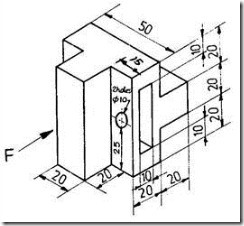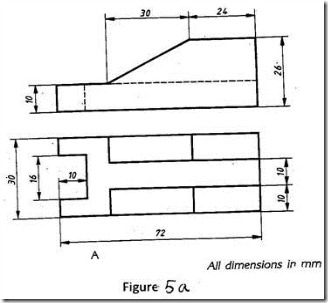### Engineering Graphics–January 2010 Question Paper

Anna University

B.E./B.Tech. DEGREE EXAMINATIONS, JANUARY 2010

Regulations 2008

First Semester

Common to all branches

GE2111 ENGINEERING GRAPHICS

Time: Three Hours

Maximum: 100 Marks

(5 x 20 = 100 Marks)

1. (a) Draw a hyperbola when the distance between its focus an d directrix is 50 mm and eccentricity is 3/2. Also draw the tangent an d normal at a point 25 mm from the directrix. (20)

OR

1. (b) Make free hand sketches of front, top and right side views of the 3D object shown below : (20)Fig. 1.(b)

2. (a) A line PQ has its end P, 10 mm above th e HP and 20 mm in front of t he VP. The end Q is 35 mm in front of t he VP. The front view of t he line measures 75 mm. The distance between th e end projectors is 50 mm. Draw the projections of t he line an d find its true length and its true inclinations with th e VP and HP. (20)

OR

2. (b) Draw th e projections of a circle of 70 mm diameter resting on t he H.P. on a point A of t he circumference. The plane is inclined to the H.P. such that th e top view of it i s an ellipse of minor axis 40 mm. The top view of t he diameter, through the point A is making an angle of 45 with t he V.P. Determine the inclination of the plane with th e H.P. (20)

3. (a) An equilateral triangular prism 20 mm side of base a nd 50 mm long rests with one of its shorter edges on HP such that the rectangular face containing th e edge on which the prism rests is inclined at 30 to H.P. The shorter edge resting on HP is perpendicular to VP. (20)

OR

3. (b) A square pyramid of base 40 mm a nd axis 70 mm long has one of its triangular faces on VP a nd th e edge of base contained by that face perpendicular to HP. Draw its projections. (20)

4. (a) A hexagonal prism of side of base 35 mm and axis length 55 mm rests with its base on H.P such that two of the vertical surfaces ar e perpendicular to V.P. It is cut by a plane inclined at 50 to H.P a nd perpendicular to V.P an d passing through a point on the axis at a distance 15 mm from th e top. Draw its front view, sectional top view and true shape of section. (20)

OR

4. (b) Draw the development of the lateral surface of the lower portion of a cylinder of diameter 50 mm a nd axis 70 mm. The solid is cut by a section plane inclined at 40 to HP and perpendicular to VP an d passing through the midpoint of the axis. (20)

5. (a) Draw th e isometric projection of t he object from th e views shown in Figure 5(a). (20)OR

5. (b) Draw the perspective projection of a cube of 25 mm edge, lying on a face on th e ground plane, with an edge touching the picture plane and all vertical faces equally inclined to th e picture plane. The station point is 50 mm in front of the picture plane, 35 mm above th e ground plane and lies in a central plane which is 10 mm to t he left of the center of the cube. (20)